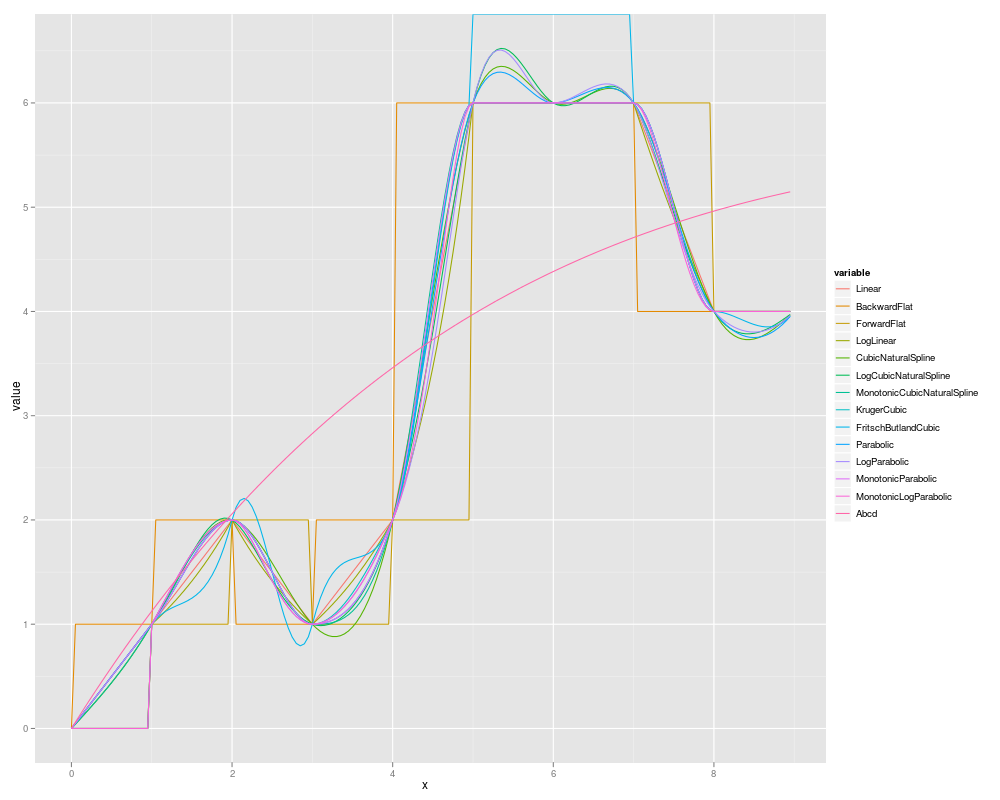Interpolation in QuantLib¶

See for example 1 for a discussion of interpolation in finance. See also our QuantLib calculator for curve interpolation here http://quantpanel.bnikolic.co.uk/InterpolatedCurve.

One dimensional interpolation¶

The following interpolation types are available in the QuantLib add-in:

• BackwardFlat

• ForwardFlat

• Linear

• LogLinear

• CubicNaturalSpline

• LogCubicNaturalSpline

• MonotonicCubicNaturalSpline

• KrugerCubic

• FritschButlandCubic

• Parabolic

• LogParabolic

• MonotonicParabolic

• MonotonicLogParabolic

• Abcd

An interpolation can be constructed by calling the qlInterpolation function and then used by calling the qlInterpolationInterpolate function.

Below is an example plot of 10 data points interpolated using the various types of interpolation:Two dimensional interpolation¶

The QuantLib add-in provides two types of two dimensional interpolation:

• BiLinear

• BiCubic

Two dimensional interpolation objects are created using the qlInterpolation2D function and can then be used to calculate an estimated value by using the qlInterpolation2DInterpolate function.

1

http://www.finmod.co.za/Hagan_West_curves_AMF.pdf